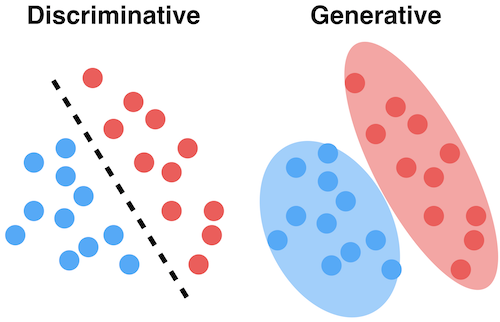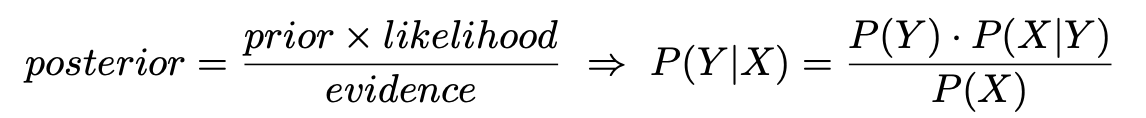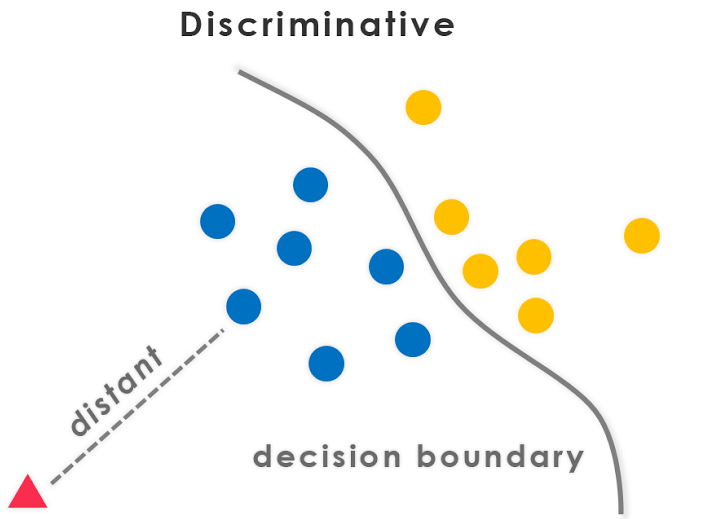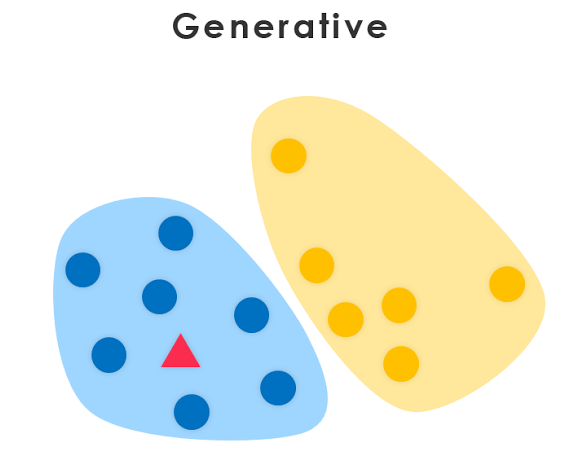This article was published as a part of the Data Science Blogathon

## Introduction

In today’s world, Machine learning becomes one of the popular and exciting fields of study that gives machines the ability to learn and become more accurate at predicting outcomes for the unseen data i.e, not seen the data in prior. The ideas in Machine learning overlaps and receives from Artificial Intelligence and many other related technologies. Today, machine learning is evolved from Pattern Recognition and the concept that computers can learn without being explicitly programmed to performing specific tasks. We can use the Machine Learning algorithms(e.g, Logistic Regression, Naive Bayes, etc) to

• Recognize spoken words,
• Data Mining, and
• Build applications that learn from data, etc.

And the improvement of these algorithms in terms of accuracy increases over time.Image Source:

Machine learning models can be classified into two types of models – Discriminative and Generative models. In simple words, a discriminative model makes predictions on the unseen data based on conditional probability and can be used either for classification or regression problem statements. On the contrary, a generative model focuses on the distribution of a dataset to return a probability for a given example.Image Source:

We as a human can adopt any of the two different approaches to machine learning models while learning an artificial language. These two models have not previously been explored in human learning. However, it is related to known effects of causal direction, classification vs. inference learning, and observational vs. feedback learning. So, In this article, our focus is on two types of machine learning models – Generative and Discriminative, and also see the importance, comparisons, and differences of these two models.

• Problem Formulation for Understanding Discriminative and Generative Models
• What are Discriminant Models in Machine Learning?
• What are Generative Models in Machine Learning?
• Difference between Discriminative and Generative Models
• Comparison between Discriminative and Generative Models

## Problem Formulation

Suppose we are working on a classification problem where our task is to decide if an email is a spam or not spam based on the words present in a particular email. To solve this problem, we have a joint model over

• Labels: Y=y, and
• Features: X={x1, x2, …xn}

Therefore, the joint distribution of the model can be represented as

` p(Y,X) = P(y,x1,x2…xn)`

Now, our goal is to estimate the probability of spam email i.e, P(Y=1|X). Both generative and discriminative models can solve this problem but in different ways.

Let’s see why and how they are different!

### The approach of Generative Models

In the case of generative models, to find the conditional probability P(Y|X), they estimate the prior probability P(Y) and likelihood probability P(X|Y) with the help of the training data and uses the Bayes Theorem to calculate the posterior probability P(Y |X):### The approach of Discriminative Models

In the case of discriminative models, to find the probability, they directly assume some functional form for P(Y|X) and then estimate the parameters of P(Y|X) with the help of the training data.

## What are Discriminative Models?

The discriminative model refers to a class of models used in Statistical Classification, mainly used for supervised machine learning. These types of models are also known as conditional models since they learn the boundaries between classes or labels in a dataset.

Discriminative models (just as in the literal meaning) separate classes instead of modeling the conditional probability and don’t make any assumptions about the data points. But these models are not capable of generating new data points. Therefore, the ultimate objective of discriminative models is to separate one class from another.

If we have some outliers present in the dataset, then discriminative models work better compared to generative models i.e, discriminative models are more robust to outliers. However, there is one major drawback of these models is the misclassification problem, i.e., wrongly classifying a data point.Image Source:

### Mathematical things involved in Discriminative Models

‌Training discriminative classifiers involve estimating a function f: X -> Y, or probability P(Y|X)

• Assume some functional form for the probability such as P(Y|X)
• With the help of training data, we estimate the parameters of P(Y|X)

### Some Examples of Discriminative Models

• ‌Logistic regression
• Scalar Vector Machine (SVMs)
• ‌Nearest neighbor
• Conditional Random Fields (CRFs)
• Decision Trees and Random Forest

## What are Generative Models?

Generative models are considered as a class of statistical models that can generate new data instances. These models are used in unsupervised machine learning as a means to perform tasks such as

• Probability and Likelihood estimation,
• Modeling data points,
• To describe the phenomenon in data,
• To distinguish between classes based on these probabilities.

Since these types of models often rely on the Bayes theorem to find the joint probability, so generative models can tackle a more complex task than analogous discriminative models.

So, Generative models focus on the distribution of individual classes in a dataset and the learning algorithms tend to model the underlying patterns or distribution of the data points. These models use the concept of joint probability and create the instances where a given feature (x) or input and the desired output or label (y) exist at the same time.

These models use probability estimates and likelihood to model data points and differentiate between different class labels present in a dataset. Unlike discriminative models, these models are also capable of generating new data points.

However, they also have a major drawback – If there is a presence of outliers in the dataset, then it affects these types of models to a significant extent.Image Source:

### Mathematical things involved in Generative Models

‌Training generative classifiers involve estimating a function f: X -> Y, or probability P(Y|X):

• Assume some functional form for the probabilities such as P(Y), P(X|Y)
• With the help of training data, we estimate the parameters of P(X|Y), P(Y)
• Use the Bayes theorem to calculate the posterior probability P(Y |X)

### Some Examples of Generative Models

• ‌Naïve Bayes
• Bayesian networks
• Markov random fields
• ‌Hidden Markov Models (HMMs)
• Latent Dirichlet Allocation (LDA)
• Autoregressive Model

## Difference between Discriminative and Generative Models

Let’s see some of the differences between Discriminative and Generative Models.

### Core Idea

Discriminative models draw boundaries in the data space, while generative models try to model how data is placed throughout the space. A generative model focuses on explaining how the data was generated, while a discriminative model focuses on predicting the labels of the data.

### Mathematical Intuition

In mathematical terms, a discriminative machine learning trains a model which is done by learning parameters that maximize the conditional probability P(Y|X), while on the other hand, a generative model learns parameters by maximizing the joint probability of P(X, Y).

### Applications

Discriminative models recognize existing data i.e, discriminative modeling identifies tags and sorts data and can be used to classify data while Generative modeling produces something.

Since these models use different approaches to machine learning, so both are suited for specific tasks i.e, Generative models are useful for unsupervised learning tasks while discriminative models are useful for supervised learning tasks.

### Outliers

Generative models have more impact on outliers than discriminative models.

### Computational Cost

Discriminative models are computationally cheap as compared to generative models.

## Comparison between Discriminative and Generative Models

Let’s see some of the comparisons based on the following criteria between Discriminative and Generative Models:

• Performance
• Missing Data
• Accuracy Score
• Applications

### Based on Performance

Generative models need fewer data to train compared with discriminative models since generative models are more biased as they make stronger assumptions i.e, assumption of conditional independence.

### Based on Missing Data

In general, if we have missing data in our dataset, then Generative models can work with these missing data, while on the contrary discriminative models can’t. This is because, in generative models, still we can estimate the posterior by marginalizing over the unseen variables. However, for discriminative models, we usually require all the features X to be observed.

### Based on Accuracy Score

If the assumption of conditional independence violates, then at that time generative models are less accurate than discriminative models.

### Based on Applications

Discriminative models are called “discriminative” since they are useful for discriminating Y’s label i.e, target outcome, so they can only solve classification problems while Generative models have more applications besides classification such as,

• Samplings,
• Bayes learning,
• MAP inference, etc.

## Self Learning Resource

If you want to go into the deep concepts about Discriminative and Generative Models, then read the following paper by Professor Andrew Ng.

Generative and Discriminative Models

## Other Blog Posts by Me

You can also check my previous blog posts.

Previous Data Science Blog posts.

Here is my Linkedin profile in case you want to connect with me. I’ll be happy to be connected with you.

## Email

For any queries, you can mail me on Gmail.

## End Notes